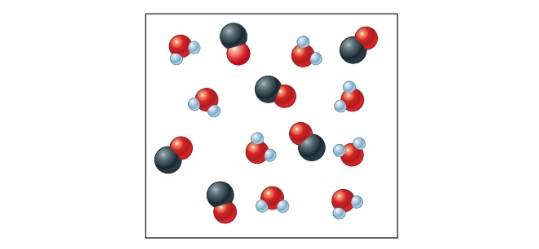Chapter 17, Problem 84AP### Introductory Chemistry: A Foundati...

9th Edition
Steven S. Zumdahl + 1 other
ISBN: 9781337399425

#### Solutions

Chapter
Section### Introductory Chemistry: A Foundati...

9th Edition
Steven S. Zumdahl + 1 other
ISBN: 9781337399425
Textbook Problem
3 views

# . Consider the following reaction at some temperature: H 2 O ( g ) + CO ( g ) ⇌ H 2 ( g ) + CO 2 ( g ) K = 2 .0 Some molecules of H2O and CO are placed in a 1.0-L container as shown below.When equilibrium is reached, how many molecules of H2O, CO, H2, and CO2are present? Do this problem by trial and error—that is, if two molecules of CO react, is this equilibrium: if three molecules of CO react, is this equilibrium: and so on.

Interpretation Introduction

Interpretation:

The molecules of H2O,  CO,  H2 and CO2 present at the equilibrium for the given reaction are to be stated.

Concept Introduction:

The equilibrium constant K is defined as the ratio of the amounts of reactants and products. It relates the concentration of chemical substances involved in a chemical reaction at the time of equilibrium. It depends on the temperature of a chemical reaction.

Explanation

The given chemical reaction is,

H2Og+COgH2g+CO2g   K=2.0

An expression for the equilibrium constant of this reaction is,

K=H2CO2H2OCO

It is given that some molecules of H2O and CO are placed in a 1.0 L container as shown below.

Figure 1

The system contains eight molecules of H2O and six molecules of CO in the container. The system comes to its equilibrium position when K=2.0. Both types of reactant lost same number of molecules when they are mixed as reaction uses the same number of each. Let assumes that number be x.

In the given reaction, the system at equilibrium contains 8x reactant molecules of H2O and 6x reactant molecules of CO. Also, the products gained x molecules of  H2 and CO2 each. Therefore, substitute the values of all concentrations and equilibrium constant in the above equilibrium expression.

2.0=x×x8x6x2.0=x2x214x+48

Simplify the above quadratic equation by trial and error method. Here, x represents the number of molecules that are converted from each reactant. Substitute x=2 in the above expression.

2

### Still sussing out bartleby?

Check out a sample textbook solution.

See a sample solution

#### The Solution to Your Study Problems

Bartleby provides explanations to thousands of textbook problems written by our experts, many with advanced degrees!

Get Started